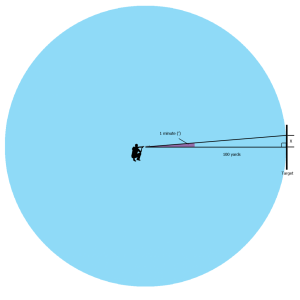# Minute of Angle

## Minute of Angle

Obviously, at times you will need to alter where you bullet hits the target, to allow for changes in wind, or proximity to the target.

Wind can move a bullet in flight significantly, and distance from a target will alter the trajectory, and as such the point of impact.

To work out how much you need to adjust you sight settings you need to know how much a minute of angle - (which is used on scopes and sights) will move a bullet at a certain distance.

You can do this by using some simple trigonometry.100 yards = 3600 inches.

1 minute = 1/60th of a degree

To work out the distance x

In this case:

Tan 1’ = x/3600

As 1’ = 1/60 degrees

Tan (1/60) =   x/3600

which rearranges as  x = 3600 Tan(1/60)

Tan (1/60) = 2.9 x10-4

So x = 3600 [2.9 x10-4]

Therefore x= 1.047 “

1 Minute of angle at 100 yards = 1.047 inches.

At 900 yards it is 9.423 inches etc.

This can easily be rounded down to 1 inch at 100 yards.

1 minute of angle moved on the scope will move the bullet 1 inch for every 100 yards of target distance.

NRA targets are scaled so that as the distance increases the size of the target also increases; the bull ring therefore is set at a diameter scaled to 2 minutes of angle.

However, you must remember that the bullet's trajectory will not follow a straight path, but will drop the further it travels due to the combined effects of gravity and air resistance.To allow for this you must bring your scope/sights onto target – (see 'Approximate Windage Allowance and Elevation' slide show in Multimedia), then smaller adjustments will be equally scaled for all NRA targets (e.g. 1 MOA at 600 yards will have the same effect on bullet placement on the target  as at 1000 yards).